# Problem 53130. Easy Sequences 55: "Ugly" Rectangles?

A positive integer n is called a regular number, if and only if there exist a non-negative integer k, such that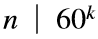. For some reason, such a number is also refered to as an ugly number. Below are the first few regular numbers: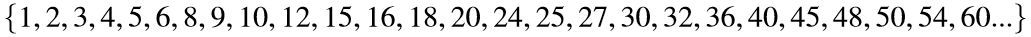We say that an integer-sided rectangle is a "regular" rectangle if its area is a regular number. In the figure below, the red-colored rectanges are regular, while the blue ones are not.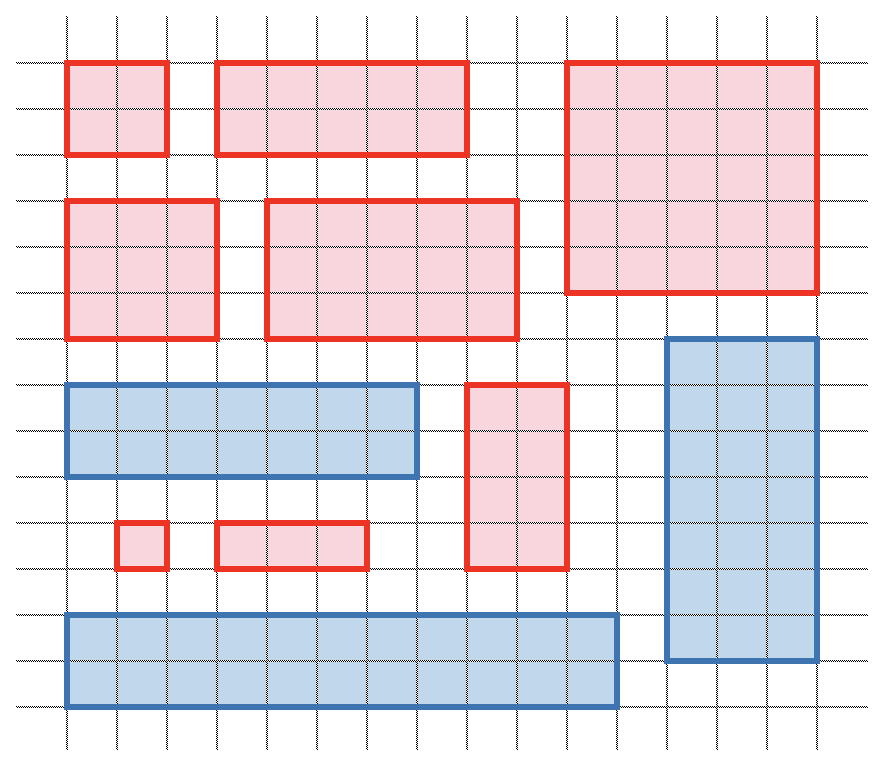All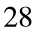regular rectangles whose areas are less than equal to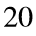are listed below: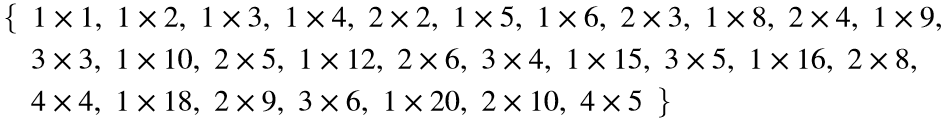And their total area is: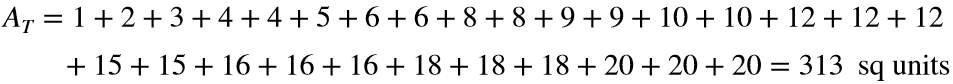For a given area limit a, find the total area of all regular rectangles with areas less than equal a.

### Solution Stats

66.67% Correct | 33.33% Incorrect
Last Solution submitted on Jul 02, 2023

### Community Treasure Hunt

Find the treasures in MATLAB Central and discover how the community can help you!

Start Hunting!